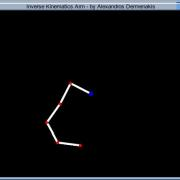# Inverse Kinematics (IK) Tutorial with Sample/Source Code in C++ with OpenGLThis tutorial provides a simplistic implementation of inverse kinematics (IK). It describes how you can construct a linked structure with N joints. The explanation of the tutorial is for N dimensions (basically we are interested in 2D and 3D) and the sample code provided below has a 2D implementation. The 3D implementation is left for you in order to practice smiley. The sample code is written in C++, uses Eigen for the mathematics and OpenGL with GLUT for rendering.

The sections below will take you step by step through the inverse kinematics mathematics and refer to the sample code.

This tutorial assumes familiarity with C++, OpenGL, Linear Algebra and Calculus (Quite incense in math but that’s how computer graphics are smiley).

Binaries Win32 Binaries

Source Code Before compiling make sure you have glut32 installed on your system!!!

For setting up glut with VS2010 consult this link.

Cross Platform Source Code with CMake and VS2010 project(Inverse Kinematics IK)